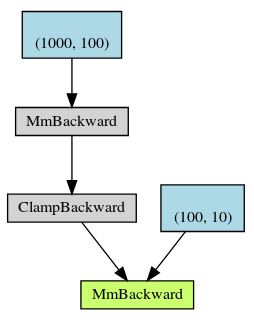# Understanding where gradients are stored in backward

I have this simple network.which is created from these lines:

``````x = torch.randn(N, D_in, device=device)
y = torch.randn(N, D_out, device=device)

w1 = torch.randn(D_in, H, device=device, requires_grad=True)
w2 = torch.randn(H, D_out, device=device, requires_grad=True)

y_pred = x.mm(w1).clamp(min=0).mm(w2)
``````

Using autograd and chain-rule, the gradients are generated from root to the leaves (here I have w1 and w2 whose requires_grad == true).

So my question is what about the intermediate “MmBackward” and “ClampBackward”. Shouldn’t we somehow store the gradients somewhere there to be used when calculating gradient of w1? If yes how can I access them?

I tried to look at “Functions.h” in generated folder but I believe grad is not an attribute of functions.

1 Like

Any thoughts/pointers/ambiguities?

they are initially freed during the backward operation for efficiency. You can use `loss.backward(retain_graph=True)` in order to keep the computation graph, however at this precise moment I am not sure if you can access the gradient of the operation. Maybe you can take a look at the documentation on the autograd package.

You’re right, but aren’t they freed after autograd finishes its job in backward? If I am not mistaken autograd uses these intermediate derivatives to calculate the gradient in leaves. So I think we can access them somewhere in c-sources.

Yeah maybe I have to look at autograd to see if I can find something there.

I think they are freed during the backward call (at least it can be done that way).

Derivatives are basically transposed matrix operations for fully connected and transposed convolutions for convolution operations. So as you go back to the initial layer you can just compute the operation and free the memory. Anyway I am not pretty sure how autograd works but I think that yes, they should be some place in the c-code where the value is momentarily stored, maybe @ptrblck or @albanD now some details on this.

As @smth explains here gradients non-leaf gradients are not retained by default to save memory. You can use the hooks mentioned in his post or call `.retain_grad()` on the particular intermediate results:

``````N, D_in, D_out, H = 1, 10, 1, 5
device = 'cpu'

x = torch.randn(N, D_in, device=device)
y = torch.randn(N, D_out, device=device)

w1 = torch.randn(D_in, H, device=device, requires_grad=True)
w2 = torch.randn(H, D_out, device=device, requires_grad=True)

x_mm1 = x.mm(w1)